# Divisor sum function

This article defines an arithmetic function or number-theoretic function: a function from the natural numbers to a ring (usually, the ring of integers, rational numbers, real numbers, or complex numbers).
View a complete list of arithmetic functions

## Definition

Letbe a natural number. The divisor sum function of, denotedor, is defined in the following equivalent ways:

1.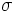is the Dirichlet product of the identity functionon the natural numbers and the all-one function: the function sending every natural number to.
2. We have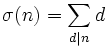.

### Formula in terms of prime factorization

Suppose we have:,

where theare distinct prime divisors of. Then: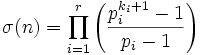.

Equivalently, the ratio is given by: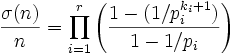.

## Behavior

### Lower bound

For any,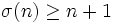. Equality is achieved if and only ifis prime. Further,is the lowest, in relative terms, for primes. In particular, excluding the case, the fractionachieves a strict minimum-so-far at every prime, and nowhere else.

Thus, we have: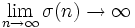,

and: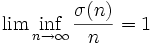,

### Upper bound

Gronwall's theorem asserts that: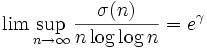where all the logarithms are natural logarithms andis the Euler-Mascheroni constant.

A closely related result is Robin's theorem, which states that the Riemann hypothesis is equivalent to the statement that for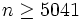, the ratio is always strictly less than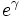.

### Logarithmic ratio

We have: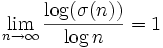.

Moreover, this approach is from the positive side, sincefor all.

## Summatory function and average value

### Summatory function

The summatory function of this function is termed the divisor sum summatory function, and is defined as:.

It is equivalent to the following: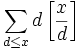.

In other words, it is the sum, over all numbers less than or equal to, of the largest multiple of that number less than or equal to. Note that this summatory function is bounded from above byand from below by.

### Average value

Given any positive real, consider the ratio: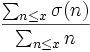.

This ratio is bounded from below byand from above by.

## Relation with other arithmetic functions

### Generalizations

• Divisor power sum function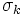is the sum of thepowers of all the positive divisors. The divisor sum function is the divisor power sum function. Also related is the divisor count function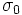, which is also denotedor.

### Other similarly defined functions

• Euler phi-function is defined as the number of integers less than or equal tothat are relatively prime to.
• Dedekind psi-function is also closely related.

### Relations expressed in terms of Dirichlet products

•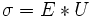:is the Dirichlet product of the identity function and the all ones function.
•: The Dirichlet product ofand the Mobius function is the identity function. Note that this is the [[Mobius inversion formula applied to the previous statement; equivalently, it is obtained by multiplying both sides of the previous equation by.
•: The Dirichlet product ofand the Euler phi-function equals the Dirichlet product of the identity function with itself, which in turn is the (pointwise) product of the identity function and the divisor count function.

### Inequalities

•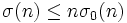, with equality iff: The sum of all divisors is less than or equal totimes the number of divisors. This follows from the obvious observation that every divisor ofis less than or equal to.
•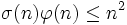, with equality iff: This can be proved, for instance, using the formula for both functions in terms of the prime factorization. There are also combinatorial proofs.

## Relation with properties of numbers

• Prime number: A natural numbersuch that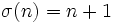.
• Perfect number: A natural numbersuch that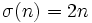.
• Quasiperfect number: A natural numbersuch that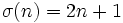.
• Abundant number: A natural numbersuch that.
• Deficient number: A natural numbersuch that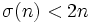.
• Superabundant number: A natural numberthat is a strict maximum-so-far for.

## Properties

### Multiplicativity

This arithmetic function is a multiplicative function: the product of this function for two natural numbers that are relatively prime is the value of the function at the product.
View a complete list of multiplicative functions

The divisor sum function is multiplicative: in other words, ifandare relatively prime positive integers, then:.

This can be proved in a number of ways. Apart from a direct proof, it also follows from the fact that the divisor sum function is a Dirichlet product of two multiplicative functions. For full proof, refer: Divisor sum function is multiplicative

### Complete multiplicativity

NO: This arithmetic function is not a completely multiplicative function: in other words, the product of the values of the function at two natural numbers need not equal the value at the product.

The divisor sum function is not a completely multiplicative function. In other words, there exist natural numberssuch that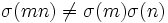. In fact, ifandare not relatively prime,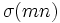is not the product ofand.

### Preservation of divisibility

NO: This arithmetic function is not a divisibility-preserving function: it does not preserve divisibility.

Ifdivides, it is not necessary thatdivides.

## Algebraic significance

The divisor sum functioncomes up in the following algebraic contexts:

• It is the sum of the sizes of all subgroups of a cyclic group of order: Thus, the ratio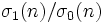is the average size of a subgroup.